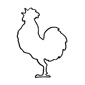# point helper per particle.

I'm trying to run through the selected particle system and create a point helper for each particle in the system. The problem I'm running into is that it creates duplicates.

```pf = \$ -- select pflow source
range = #(0, 30)

fn fnAddKey Obj val curTime =
(
with animate on
(
objTrack = Obj.pos.x = 5
)
)

for t = range to range do
(
MatEditor.Close()
local pPos = undefined
local flasherObj
sliderTime = t
count = pf.NumParticles()

temp = for i = 1 to count do
(
pf.particleIndex = i
pPos = pf.particlePosition
flasherObj = point wirecolor:yellow pos:pPos
)
)```

## Comment viewing options### This might help. I re-wrote

This might help. I re-wrote the code you originally put in but i put a test on it to only create a new point if one does not exist.

```pf = \$ -- select pflow source
range = #(0, 30)
ptArray = #()
ptTest = 0

(
with animate on
(
at time curTime
(
Obj.position = [5 ,obj.position.y, obj.position.z]
)
)
)

--call outside of loop once
MatEditor.Close()
for t = range to range do
(
count = pf.NumParticles()

for i = 1 to count do
(
pf.particleIndex = i
ptId = pf.particleId
if ptID > ptTest then
(
pPos = pf.particlePosition
newPt = point wirecolor:yellow pos:pPos
append ptArray newPt
ptTest = ptId
) else (
curPt = ptArray[ptID]
)
)
)```### Kinda

It's kind of what I was looking for. I want to create a point helper each time a new particle is created. And at the exact time that the particle is birthed I want to animate that halper moving in the z axis "x" amount of units.

I've attached the match file to help get things started.
If you open the file and run the script I posted in the main part of this post youll see the problem.

AttachmentSize
04test.max 220 KB

John Martini
Digital Artist
http://www.JokerMartini.com (new site)### You are having the loop which

You are having the loop which creates the particle be called 30 times causing you to make new point helpers since you have a loop running with in another loop. Also be careful with the NumParticles method since that only gives you the number of particles currently at that frame. If you know how many particles you are going to have i would just type it in. I'm also not sure what you are trying to accomplish with this code but this is what i got out of it.

```pf = \$		-- select pflow source
ptArray = #() 	-- Array to hold your points
range = #(0, 30)-- Fram range
ptAmount = pf.NumParticles()	--Number of particles currently on this frame

fn fnAddKey curTime ptNum pfVar ptAr=
(
disablesceneredraw()
with animate on
(
for pt = 1 to ptNum do
(
pfVar.particleIndex = pt
pPos = pfVar.particlePosition
at time curTime
ptAr[pt].position = [5 , pPos.y, pPos.z]
)
)
enablesceneredraw()

MatEditor.Close()-- No need to call this inside the Loop
max create mode

for pt = 1 to ptAmount do
(
ptVar = point wirecolor:yellow
append ptArray ptVar
)

for timeVar = range to range do
(